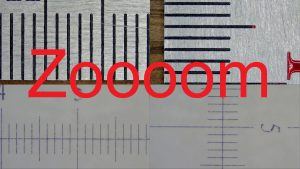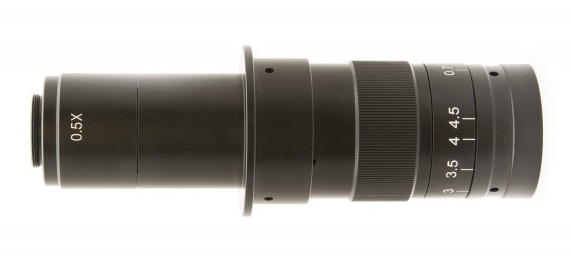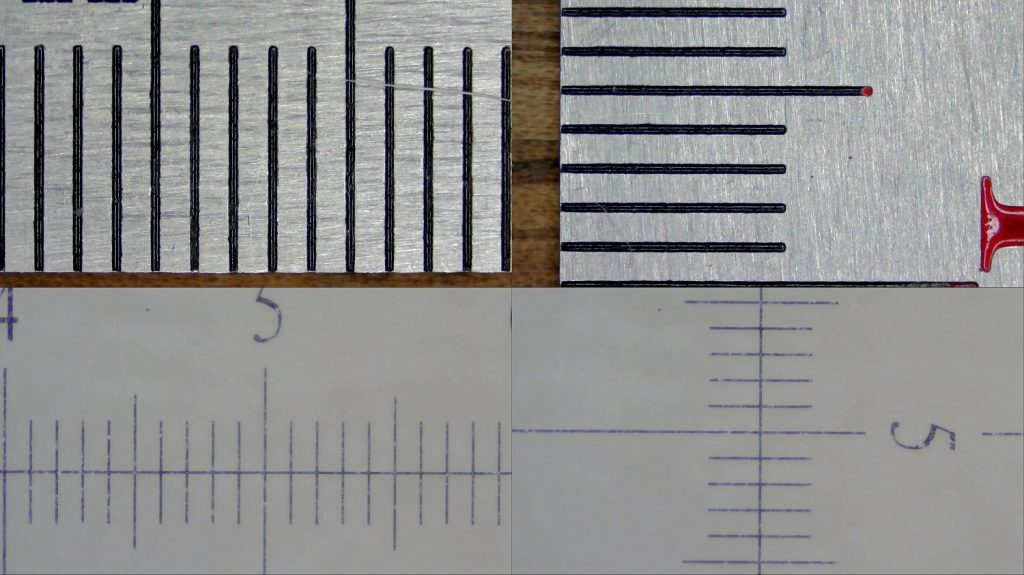Browse over 10,000 Electronics Projects

# Measuring minimal and maximal microscope magnification and zoom ratioMagnification ratio is one of many parameters to describe optical instrument – microscope in this case. Magnification ratio usually comes along with zoom range. These terms are often being misused. In this post I will measure zoom range and magnification ratio of monocular video microscope lens and stereo inspection microscope (Konus #5424) head.

Despite being different kind of optical instruments they have similar magnification ratios, so it’s fair to compare them.

### Measurement

Let’s mount C1 camera on a lens and take some pictures of a known object at minimal and maximal magnification.Two different rulers will be used:

• Larger one is 50cm steel ruler
• Smaller one is microscope slide 5mm with 0.01mm marksI will use these pictures to measure view field dimensions. Also to be noted that video microscope uses 0.5x adapter tube.

```Declared min magnification: (0.7x * 0.5) 0.35x -> 13mm(H) x 7.3mm(V)
Declared max magnification: (4.5x * 0.5) 2.25x -> 2mm(H) x 1.1mm(V)```

### Math – zoom ratio

Quickly calculate diagonal viewable image size at min and max magnification:

```SQRT(13^2 + 7.3^2) = 14.9mm
SQRT(2^2 + 1.1^2) = 2.3mm```

And now we can find zoom ratio:

```Theoretical: (4.5*0.5) / (0.7*0.5) = 6.4x
Zoom ratio: 14.9 / 2.3 = 6.5x```

So stated magnification ratio is pretty legit. Good to know that manufacturer does not lie.

For reference I also measured trinocular microscope viewable image size at minimal and maximal magnification ratio. Please note that this time I measured rulers by eye and projected image is disc instead of being rectangular.

```Declared min magnification 0.7x: D=33mm
Declared max magnification 4.5x: D=4.5mm
Zoom ratio: 33/4.5 = 7.3x```

While specified parameters are the same magnification range is slightly wider than previous optical instrument.

### Math – magnification

While we can’t measure stereo microscope magnification (don’t have projected image dimensions), it is pretty easy to calculate this parameter for lens mounted on a camera. To calculate this parameter we need to know used camera sensor dimensions. Good to know that C1 camera has known sensor dimensions.

```Sensor size horizontal: 5.07mm(H)
Sensor size horizontal: 3.38mm(V)
Sensor size diagonal: SQRT(5.07^2 + 3.38^2) = 6.09mm
```

Also let’s take into account that camera does not use full sensor.

```Pixel dimensions: 2.2µm x 2.2µm (square pixels)
Frame size: 1920 x 1080
True frame size horizontal: 1920 * 2.2µm = 4.224mm
True frame size vertical: 1080 * 2.2µm = 2.376mm
True frame size diagonal: SQRT(4.224^2 + 2.376^2) = 4.85mm
```Now when we have source and destination image sizes let’s normalize them to have nice magnification ratio

Min magnification: 4.85 / 14.9 = 0.33 = 1:3 Max magnification: 4.85 / 2.28 = 2.12 = 2:1

Simplified animated illustration shows projected image size variations.### Compare results

To compare viewable area of both microscopes I provide you drawing.While both compared microscopes have specified range of 0.7x-4.5x they have slightly different optical parameters. At a first glance video microscope has smaller view area, but it might be deceptive conclusions. Actually being C-mount it is suitable for larger sensors. How big? It might be between 1″ and 1/2″.

Top